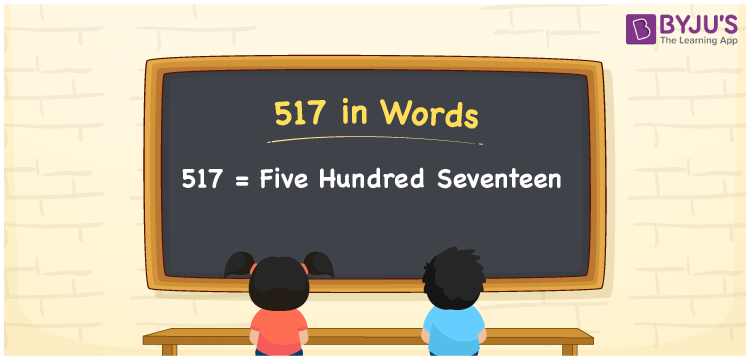# 517 in Words

517 in words is written as Five hundred seventeen. In both the International System of Numerals and the Indian System of Numerals, 517 is written as Five hundred seventeen. The number 517 is a Cardinal Number as it represents some quantity. For example, “517 tables and chairs are required”.

 517 in Words Five hundred seventeen Five hundred seventeen in Number 517

## 517 in English Words

We write 517 in English Words using the letters of the English alphabet. Therefore, we read 517 in English as “Five hundred seventeen.”## How to Write 517 in Words?

To write 517 in words, we shall use the place value chart. In the place value chart, write 5 in the hundreds, 1 in the tens, and 7 in the ones, respectively. Now let us make a place value chart to write the number 517 in words.

 Hundreds Tens Ones 5 1 7

Thus, we can write the expanded form as

5 × Hundred + 1 × Ten + 7 × One

= 5 × 100 + 1 × 10 + 7 × 1

= 500 + 10 + 7

= 517

= Five hundred seventeen.

517 is a natural number, the successor of 516 and the predecessor of 518.

517 in words – Five hundred seventeen

• Is 517 an odd number? – Yes
• Is 517 an even number? – No
• Is 517 a perfect square number? – No
• Is 517 a perfect cube number? – No
• Is 517 a prime number? – No
• Is 517 a composite number? – Yes

## Frequently Asked Questions on 517 in Words

Q1

### How to write 517 in words?

517 in words is written as Five hundred seventeen.
Q2

### How to write 517 in the International and Indian System of Numerals?

In both, the system of numerals, 517 in words, is written as Five hundred seventeen.
Q3

### What is the preceding number of 517?

The number that precedes 517 is 516.